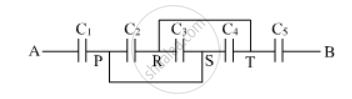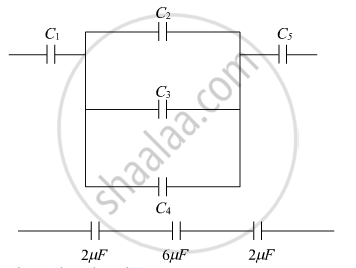Department of Pre-University Education, KarnatakaPUC Karnataka Science Class 12

# Find equivalent capacitance between A and B in the combination given below. Each capacitor is of 2 µF capacitance. - Physics

(i) Find equivalent capacitance between A and B in the combination given below. Each capacitor is of 2 µF capacitance.(ii) If a dc source of 7 V is connected across AB, how much charge is drawn from the source and what is the energy stored in the network?

#### Solution

(i)1/C_(eqv)=1/2+1/6+1/2=7/6

C_(eqv)=6/7 μF

(ii) Q=C_(eqv)V

Q=6/7×7

Q=6 " μC"

E=1/2CV^2

E=1/2×6/7×(49)

E=21 J

Concept: Capacitors and Capacitance
Is there an error in this question or solution?